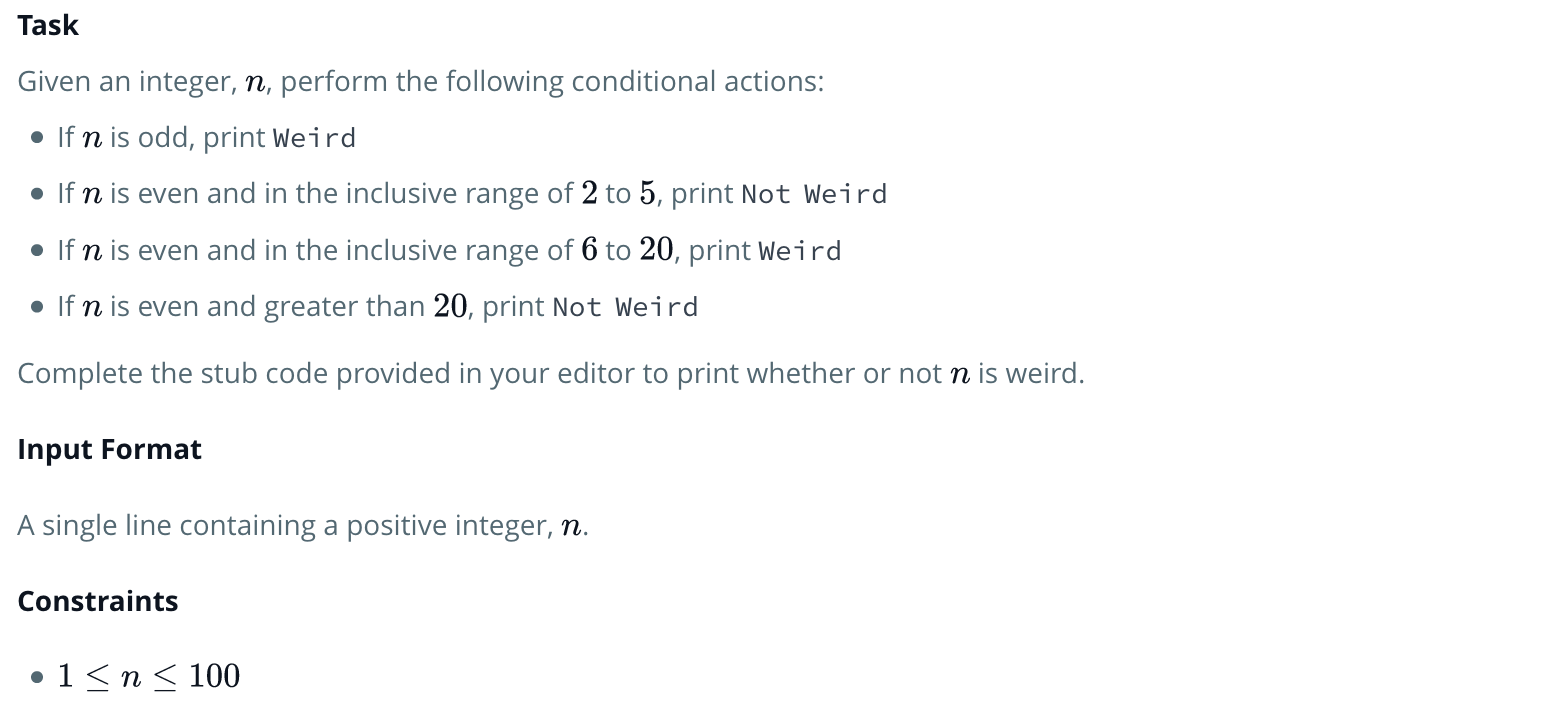# HackerRank 30 days of coding – Day3 ( Intro to Conditional Statements)Sample Input 0

``````3
``````

Sample Output 0

``````Weird
``````

Sample Input 1

``````24
``````

Sample Output 1

``Not Weird``

Solution:

```#!/bin/python

import math
import os
import random
import re
import sys

List1=[2,3,4,5]
List2=[6,7,8,9,10,11,12,13,14,15,16,17,18,19,20]

def Weird():
if N%2!=0:
print"Weird"
elif N%2==0 and N in List1:
print"Not Weird"
elif N%2==0 and N in List2:
print"Weird"
elif N%2==0 and 100>=N>20:
print"Not Weird"

if __name__ == '__main__':
N = int(raw_input())
Weird()```